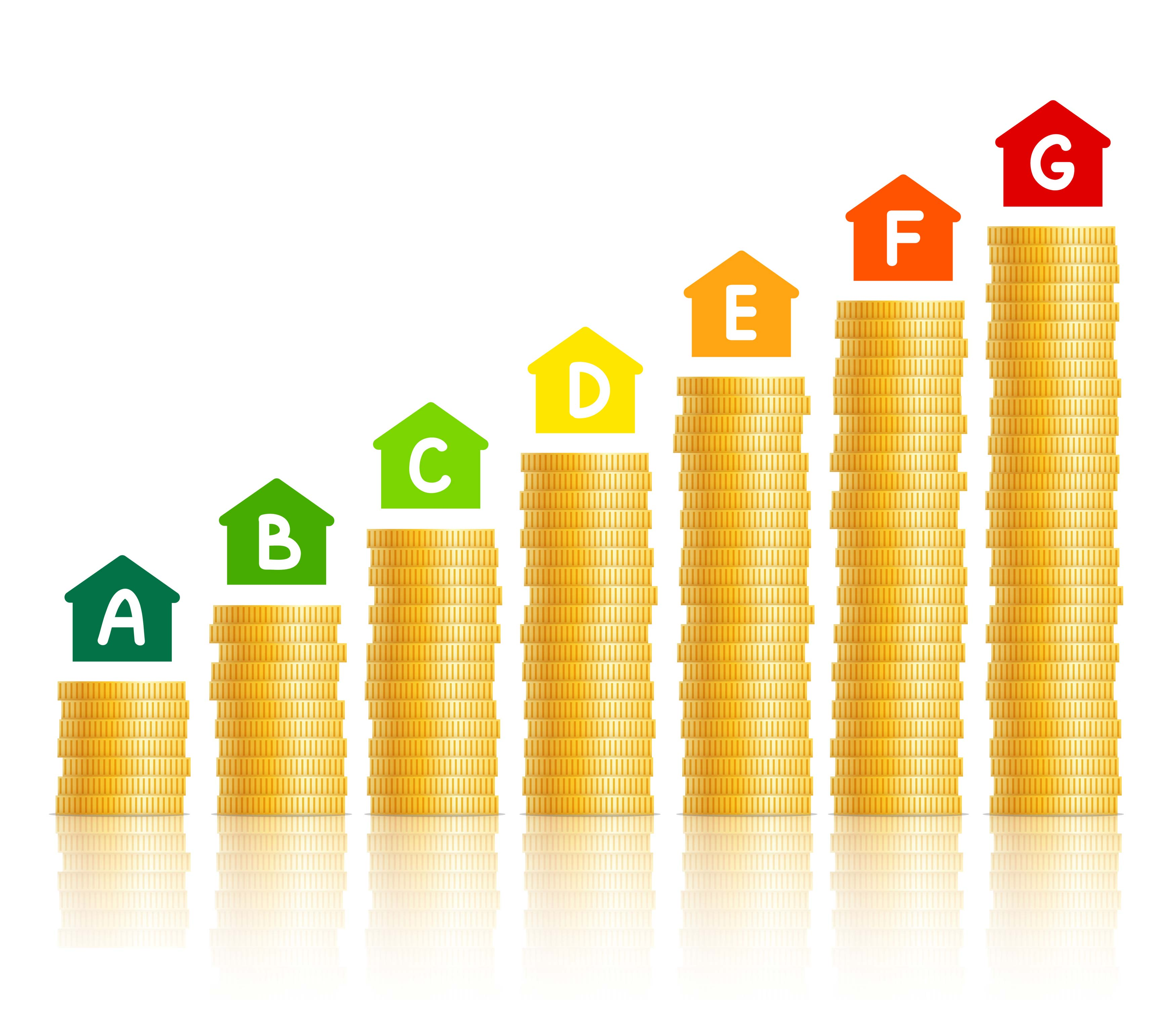What's Watt with running costsEnergy bills are a major household expense. Using energy more efficiently will help to cut the cost of your bills as well as reducing your carbon emissions. But first you need to understand ‘what’s Watt’ - that is how much electricity your appliances use. It helps to focus on appliances that use most energy and therefore cost the most to run. As a rule, those with moving parts or which produce heat use much more than those that produce light or sound.

Electricity consumption is usually measured in kilowatt hours, or kWh for short. Each kWh of electricity equates to 'one unit of electricity' for billing purposes. The cost per unit used depends on which tariff you are on. Every electrical appliance has a power rating, usually given in Watts (W) or kilowatts  (1000W =1kW). How much an appliance costs to run depends on how long it is on for. So you can work out how much an appliance costs to run by multiplying its wattage by the amount of time it’s on and then by the cost of electricity per unit (kWs x 1hr x unit rate = cost to run per hour).

For example, a 2kW appliance on for three hours uses six kWhs, or six units of electricity. On Jersey Electricity’s General Domestic Tariff of 15.68p a unit (incl) GST, that’s 94p.

• A 1kW (1,000 Watts) appliance in use for one hour = 1kWh (one unit)

• A 3kW (3,000 Watts) appliance in use for one hour = 3kWh (three units)

• A 100W light bulb in use for 10 hours = 1kWh (one unit)

To complicate matters, a higher-wattage appliance can use less energy overall than a lower-wattage one if it does its job quicker. An energy efficient dish washer, for example, may have the same or even a higher power rating than a non-energy efficient one but because it completes its cycle quicker, it uses less energy overall. Appliances rated on the label as rated ‘A’ or above are the most efficient for their size.

To compare between differently sized appliances, energy labels also now print suggested kWh usage per annum for each appliance.